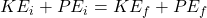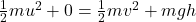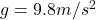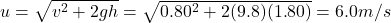## In the high jump, the kinetic energy of an athlete is transformed into gravitational potential energy without the aid of a pole. With what m

Question

In the high jump, the kinetic energy of an athlete is transformed into gravitational potential energy without the aid of a pole. With what minimum speed must the athlete leave the ground in order to lift his center of mass 1.80 m and cross the bar with a speed of 0.80 m/s?

in progress 0
3 weeks 2021-08-26T18:37:19+00:00 1 Answers 0 views 0

6.0 m/s

Explanation:

According to the law of conservation of energy, the total mechanical energy (potential, PE, + kinetic, KE) of the athlete must be conserved.

Therefore, we can write:orwhere:

m is the mass of the athlete

u is the initial speed of the athlete (at the bottom)

0 is the initial potential energy of the athlete (at the bottom)

v = 0.80 m/s is the final speed of the athlete (at the top)is the acceleration due to gravity

h = 1.80 m is the final height of the athlete (at the top)

Solving the equation for u, we find the initial speed at which the athlete must jump: